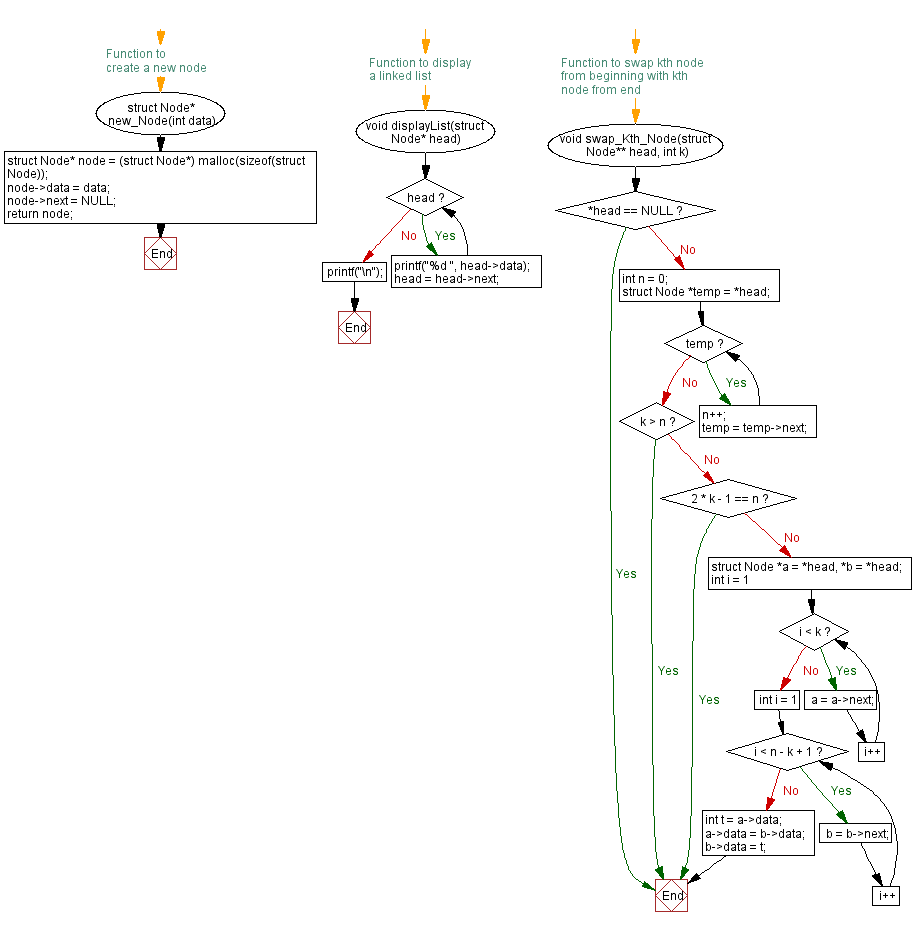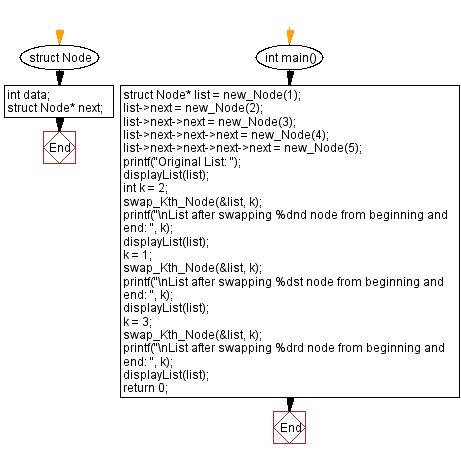﻿ C Program: Change Kth node from beginning to end in a linked list

# C Exercises: Change Kth node from beginning to end in a linked list

## C Singly Linked List : Exercise-24 with Solution

Write a C program to swap Kth node from the beginning with Kth node from the end in a singly linked list.

Sample Solution:

C Code:

``````#include<stdio.h>
#include <stdlib.h>

struct Node {
int data;
struct Node* next;
};

// Function to create a new node
struct Node* new_Node(int data) {
struct Node* node = (struct Node*) malloc(sizeof(struct Node));
node->data = data;
node->next = NULL;
return node;
}

// Function to display a linked list
}
printf("\n");
}
// Function to swap kth node from beginning with kth node from end
void swap_Kth_Node(struct Node** head, int k) {
int n = 0;
while (temp) {
n++;
temp = temp->next;
}

if (k > n) return;
if (2 * k - 1 == n) return;
for (int i = 1; i < k; i++)
a = a->next;
for (int i = 1; i < n - k + 1; i++)
b = b->next;

int t = a->data;
a->data = b->data;
b->data = t;
}

int main() {
struct Node* list = new_Node(1);
list->next = new_Node(2);
list->next->next = new_Node(3);
list->next->next->next = new_Node(4);
list->next->next->next->next = new_Node(5);

printf("Original List: ");
displayList(list);
int k = 2;
swap_Kth_Node(&list, k);
printf("\nList after swapping %dnd node from beginning and end: ", k);
displayList(list);
k = 1;
swap_Kth_Node(&list, k);
printf("\nList after swapping %dst node from beginning and end: ", k);
displayList(list);
k = 3;
swap_Kth_Node(&list, k);
printf("\nList after swapping %drd node from beginning and end: ", k);
displayList(list);
return 0;
}
```
```

Sample Output:

```Original List: 1 2 3 4 5

List after swapping 2nd node from beginning and end: 1 4 3 2 5

List after swapping 1st node from beginning and end: 5 4 3 2 1

List after swapping 3rd node from beginning and end: 5 4 3 2 1
```

Flowchart :C Programming Code Editor:

What is the difficulty level of this exercise?

﻿